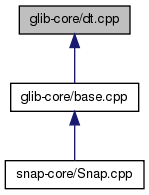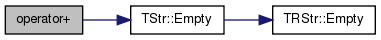SNAP Library 6.0, Developer Reference  2020-12-09 16:24:20 SNAP, a general purpose, high performance system for analysis and manipulation of large networks
dt.cpp File Reference
This graph shows which files directly or indirectly include this file:Go to the source code of this file.

## Macros

#define __TEST(from, to, len)

## Functions

TStr operator+ (const TStr &LStr, const TStr &RStr)

TStr operator+ (const TStr &LStr, const char *RCStr)

## Macro Definition Documentation

 #define __TEST ( from, to, len )
Value:
for (i = (from); i <= (to); i++) \
{ if ((i & 0xffff) == 0) printf("%d\r", i); \
r = SaveFrugalInt(p, i); s = LoadFrugalInt(p, j); \
IAssert(r == s); IAssert(i == j); IAssert(r - p == len); }
#define IAssert(Cond)
Definition: bd.h:262

Referenced by TInt::TestFrugalInt().

## Function Documentation

 TStr operator+ ( const TStr & LStr, const TStr & RStr )

Definition at line 1631 of file dt.cpp.

References TStr::Empty().

1631  {
1632  if (LStr.Empty()){return RStr;}
1633  else if (RStr.Empty()){return LStr;}
1634  else {return TStr(LStr)+=RStr;}
1635 }
Definition: dt.h:412
bool Empty() const
Definition: dt.h:491

Here is the call graph for this function:TStr operator+ ( const TStr & LStr, const char * RCStr )

Definition at line 1637 of file dt.cpp.

1637  {
1638  return TStr(LStr)+=RCStr;
1639 }
Definition: dt.h:412